Newton's Laws - Lesson 3 - Newton's Second Law of Motion

# Finding Individual Forces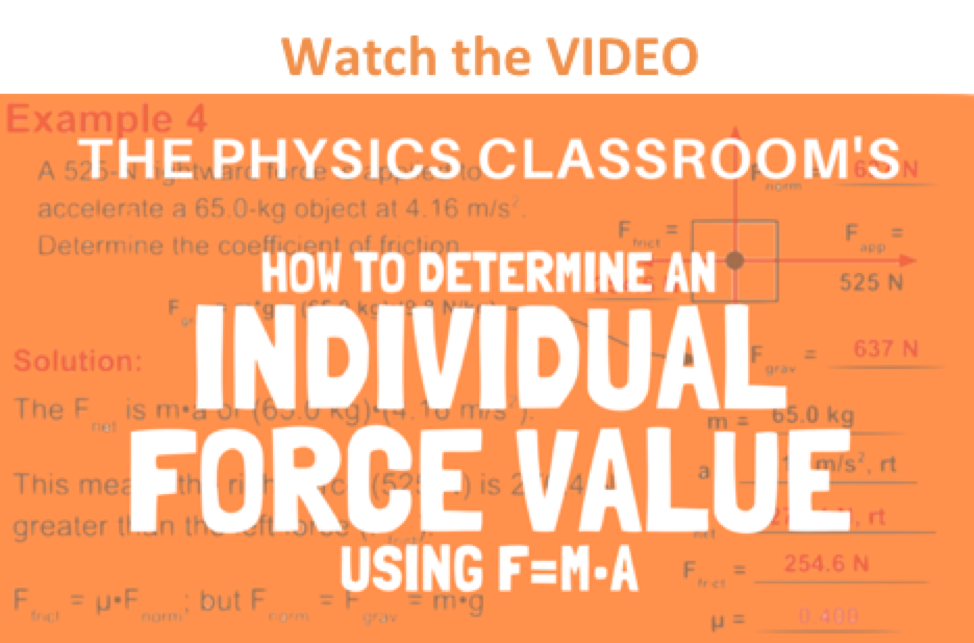As learned earlier in Lesson 3 (as well as in Lesson 2), the net force is the vector sum of all the individual forces. In Lesson 2, we learned how to determine the net force if the magnitudes of all the individual forces are known. In this lesson, we will learn how to determine the magnitudes of all the individual forces if the mass and acceleration of the object are known. The three major equations that will be useful are the equation for net force (Fnet = m•a), the equation for gravitational force (Fgrav = m•g), and the equation for frictional force (Ffrict = μ•Fnorm).

The process of determining the value of the individual forces acting upon an object involve an application of Newton's second law (Fnet=m•a) and an application of the meaning of the net force. If mass (m) and acceleration (a) are known, then the net force (Fnet) can be determined by use of the equation.

Fnet = m • a

If the numerical value for the net force and the direction of the net force is known, then the value of all individual forces can be determined. Thus, the task involves using the above equations, the given information, and your understanding of net force to determine the value of individual forces.

### Your Turn to Practice

To gain a feel for how this method is applied, try the following practice problems. The problems progress from easy to more difficult. Once you have solved a problem, click the button to check your answers.

### Practice #1

Free-body diagrams for four situations are shown below. The net force is known for each situation. However, the magnitudes of a few of the individual forces are not known. Analyze each situation individually and determine the magnitude of the unknown forces.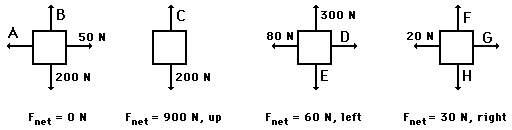### Practice #2

A rightward force is applied to a 6-kg object to move it across a rough surface at constant velocity. The object encounters 15 N of frictional force. Use the diagram to determine the gravitational force, normal force, net force, and applied force. (Neglect air resistance.)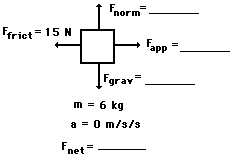### Practice #3

A rightward force is applied to a 10-kg object to move it across a rough surface at constant velocity. The coefficient of friction between the object and the surface is 0.2. Use the diagram to determine the gravitational force, normal force, applied force, frictional force, and net force. (Neglect air resistance.)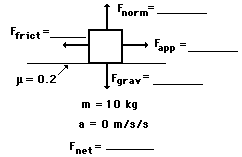### Practice #4

A rightward force is applied to a 5-kg object to move it across a rough surface with a rightward acceleration of 2 m/s/s. The coefficient of friction between the object and the surface is 0.1. Use the diagram to determine the gravitational force, normal force, applied force, frictional force, and net force. (Neglect air resistance.)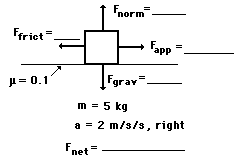### Practice #5

A rightward force of 25 N is applied to a 4-kg object to move it across a rough surface with a rightward acceleration of 2.5 m/s/s. Use the diagram to determine the gravitational force, normal force, frictional force, net force, and the coefficient of friction between the object and the surface. (Neglect air resistance.)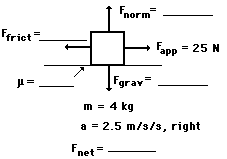A couple more practice problems are provided below. You should make an effort to solve as many problems as you can without the assistance of notes, solutions, teachers, and other students. Commit yourself to individually solving the problems. In the meantime, an important caution is worth mentioning:

Avoid forcing a problem into the form of a previously solved problem. Problems in physics will seldom look the same. Instead of solving problems by rote or by mimicry of a previously solved problem, utilize your conceptual understanding of Newton's laws to work towards solutions to problems. Use your understanding of weight and mass to find the m or the Fgrav in a problem. Use your conceptual understanding of net force (vector sum of all the forces) to find the value of Fnet or the value of an individual force. Do not divorce the solving of physics problems from your understanding of physics concepts. If you are unable to solve physics problems like those above, it is does not necessarily mean that you are having math difficulties. It is likely that you are having a physics concepts difficulty.

### We Would Like to Suggest ...Sometimes it isn't enough to just read about it. You have to interact with it! And that's exactly what you do when you use one of The Physics Classroom's Interactives. We would like to suggest that you combine the reading of this page with the use of our Force Interactive. You can find it in the Physics Interactives section of our website. The Force Interactive allows a learner to explore the effect of variations in applied force, net force, mass, and friction upon the acceleration of an object.

Visit:  Force

### Check Your Understanding

1. Lee Mealone is sledding with his friends when he becomes disgruntled by one of his friend's comments. He exerts a rightward force of 9.13 N on his 4.68-kg sled to accelerate it across the snow. If the acceleration of the sled is 0.815 m/s/s, then what is the coefficient of friction between the sled and the snow?

2. In a Physics lab, Ernesto and Amanda apply a 34.5 N rightward force to a 4.52-kg cart to accelerate it across a horizontal surface at a rate of 1.28 m/s/s. Determine the friction force acting upon the cart.

Next Section:
Jump To Next Lesson:

Follow Us